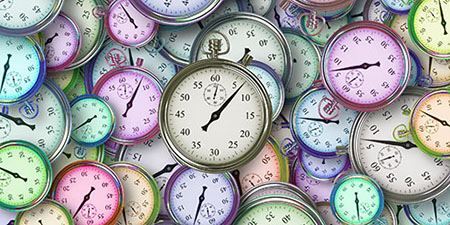# Millennium (ml) to Month (mo)

Thema Time units ﹣ Converter Millennium to Month

Here you can convert the Time unit Millennium into the unit Month and vice versa you can convert Month into Millennium. By clicking the "Swap units" icon, you will always obtain the desired conversion in the calculation result, i.e., ml to mo or mo to ml. With the following calculator you can also calculate any other Time unit.

## Info about "Millennium"

A millennium (abbreviated as ml) represents a period of one thousand years. A millennium consists of ten centuries. Like the year itself, the millennium is not part of the international system of units.

A millennium begins on 1st January of the first year (year 1) and ends on 31st December of a thousand years (year 1000). For example, the 3rd century begins on 1st January of the year 2000 and ends on 31st December, 2999. It is the 3rd millennium as there were 2 millennia before it. This is similar to the age of life: On one's 2nd birthday, one completes his 2nd year of life. From the 2nd birthday, the 3rd year of life begins.

## Info about "Month"

A month forms the time span of 28, 29, 30 or 31 consecutive days. Due to its varying length, the month is not a physical unit of time and thus not a unit of time in the international SI system of units.

To enable a general conversion (e.g., into seconds, minutes or years), the average length of the month in days is defined: Since a year comprises 12 months, a normal calendar year with 365 days results in a month length of 365 / 12 = 30.416667 days

In astronomical terms, a month is defined as the period of one complete revolution of the moon. Depending on the definition, the lunar month is a little more than 28 days on average.

## Basis for conversion Millennium (ml) to Month (mo) and vice versa

The abbreviation for the "Time unit Millennium" is ml. The abbreviation for the "Time unit Month" is mo.

## Formula for the conversion of Millennium (ml) to Month (mo) and vice versa

The calculation from Millennium to Month shall be made using the following conversion formula:

Conversion formula Millennium to Month

Determine the number of Month from Millennium

Millennium × 12000

## Formula for the conversion of Month (mo) to Millennium (ml)

The calculation from Month to Millennium shall be made using the following conversion formula:

Conversion formula Month to Millennium

Determine the number of Millennium from Month

Month × 8.3333333333333E-5

## Overview table : How many Millennium are in Month ?

Millennium ml ⇒ Month mo
0.01 ml  are  120 mo
0.02 ml  are  240 mo
0.03 ml  are  360 mo
0.04 ml  are  480 mo
0.05 ml  are  600 mo
0.06 ml  are  720 mo
0.07 ml  are  840 mo
0.08 ml  are  960 mo
0.09 ml  are  1 080 mo
0.10 ml  are  1 200 mo
0.20 ml  are  2 400 mo
0.30 ml  are  3 600 mo
0.40 ml  are  4 800 mo
0.50 ml  are  6 000 mo
0.60 ml  are  7 200 mo
0.70 ml  are  8 400 mo
0.80 ml  are  9 600 mo
0.90 ml  are  10 800 mo
1 ml  corresponds to  12 000 mo
2 ml  are  24 000 mo
3 ml  are  36 000 mo
4 ml  are  48 000 mo
5 ml  are  60 000 mo
6 ml  are  72 000 mo
7 ml  are  84 000 mo
8 ml  are  96 000 mo
9 ml  are  108 000 mo
10 ml  are  120 000 mo
20 ml  are  240 000 mo
30 ml  are  360 000 mo
40 ml  are  480 000 mo
50 ml  are  600 000 mo
60 ml  are  720 000 mo
70 ml  are  840 000 mo
80 ml  are  960 000 mo
90 ml  are  1 080 000 mo
100 ml  are  1 200 000 mo
200 ml  are  2 400 000 mo
300 ml  are  3 600 000 mo
400 ml  are  4 800 000 mo
500 ml  are  6 000 000 mo
600 ml  are  7 200 000 mo
700 ml  are  8 400 000 mo
800 ml  are  9 600 000 mo
900 ml  are  10 800 000 mo
1 000 ml  are  12 000 000 mo

## Overview table : How many Month are in Millennium ?

Month mo ⇒ Millennium ml
0.20 mo  are  0.00001 ml
0.30 mo  are  0.00002 ml
0.40 mo  are  0.00003 ml
0.50 mo  are  0.00004 ml
0.60 mo  are  0.00005 ml
0.70 mo  are  0.00005 ml
0.80 mo  are  0.00006 ml
0.90 mo  are  0.00007 ml
1 mo  corresponds to  0.00008 ml
2 mo  are  0.00016 ml
3 mo  are  0.00025 ml
4 mo  are  0.00033 ml
5 mo  are  0.00041 ml
6 mo  are  0.00050 ml
7 mo  are  0.00058 ml
8 mo  are  0.00066 ml
9 mo  are  0.00075 ml
10 mo  are  0.00083 ml
20 mo  are  0.00166 ml
30 mo  are  0.00250 ml
40 mo  are  0.00333 ml
50 mo  are  0.00416 ml
60 mo  are  0.00500 ml
70 mo  are  0.00583 ml
80 mo  are  0.00666 ml
90 mo  are  0.00750 ml
100 mo  are  0.00833 ml
200 mo  are  0.01666 ml
300 mo  are  0.02500 ml
400 mo  are  0.03333 ml
500 mo  are  0.04166 ml
600 mo  are  0.05000 ml
700 mo  are  0.05833 ml
800 mo  are  0.06666 ml
900 mo  are  0.07500 ml
1 000 mo  are  0.08333 ml

## Source information

As source for the information in the "Time units" category, we have used in particular:

## Last update on May 2, 2022

The pages of the "Time units" category were last editorially reviewed by Stefan Banse on May 2, 2022. They all correspond to the current status.

### Previous changes on April 24, 2021

• April 24, 2021: Publication of the time units converter.
• Editorial revision of all texts in this category